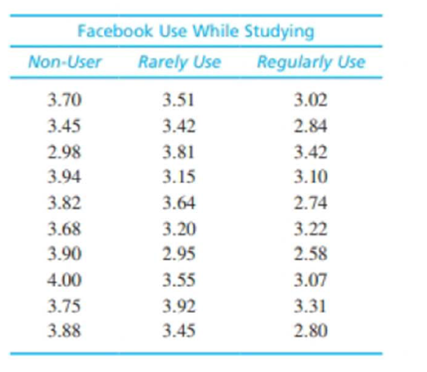Chapter 12, Problem 23PEssentials of Statistics for the B...

8th Edition
Frederick J Gravetter + 1 other
ISBN: 9781133956570

Solutions

Chapter
SectionEssentials of Statistics for the B...

8th Edition
Frederick J Gravetter + 1 other
ISBN: 9781133956570
Textbook Problem

There is some research indicating that college students who use Facebook while studying tend to have lower grades than non-users (Kirschner &. Karpinski, 2010). A representative study surveys students to determine the amount of Facebook use during the time they are studying or doing homework. Based on the amount of time spent on Facebook, students are classified into three groups and their grade point averages are recorded. The following data show: the typical pattern of results.a. Use an ANOVA with α = .05 to determine whether there are significant mean differences among the three groups.b. Compute η 2 to measure the size of the effect.c. Write a sentence demonstrating how the result from the hypothesis test and the measure of effect size would appear in a research report.

a.

To determine

To find: Whether there is any significant difference among the three groups using ANOVA with α=0.05

Explanation

Given info:

In one survey some students were surveyed for determining the amount of Facebook use during studying. According their answers, the students were classified in three groups. The grade points of each student are given.

Calculations:

The null and alternative hypotheses:

Null hypothesis:

H0:μ1=μ2=μ3

That is, all treatments have equal mean.

Alternate hypothesis:

H1:At least two means are different

Degrees of freedom:

It is known that for k number of treatments the degrees of freedom is,

df=k1.

Thus,

dfbetween=k1=21=1

and,

dfwithin=Nk=243=21

Critical value:

For α=0

b.

To determine

To Find: η2 for the given question

c.

To determine

To write: A sentence that demonstrate how a research report would present the results of the hypothesis test and the measure of effect size.

Still sussing out bartleby?

Check out a sample textbook solution.

See a sample solution

The Solution to Your Study Problems

Bartleby provides explanations to thousands of textbook problems written by our experts, many with advanced degrees!

Get Started

Explain why 2004 nickels are worth more than 100.

Mathematical Excursions (MindTap Course List)

Fill in the blanks. 3.The distance between two points P1(a, b) and P2(c, d) is _________.

Applied Calculus for the Managerial, Life, and Social Sciences: A Brief Approach

Use the given graph of f to sketch the graph of f1. 29.

Single Variable Calculus: Early Transcendentals, Volume I

True or False: Du f(a, b) = ∇(a, b) · u.

Study Guide for Stewart's Multivariable Calculus, 8th

True or False: f(x) = 10x − x2 is increasing on (4,8).

Study Guide for Stewart's Single Variable Calculus: Early Transcendentals, 8th